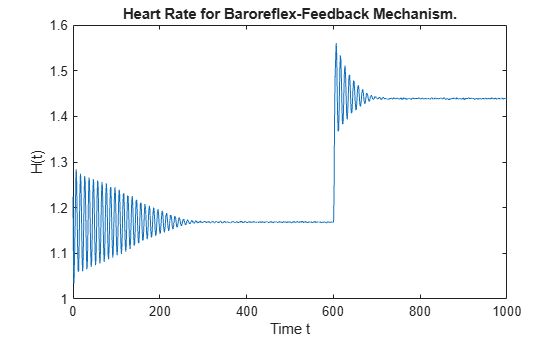Main Content

# Cardiovascular Model DDE with Discontinuities

This example shows how to use `dde23` to solve a cardiovascular model that has a discontinuous derivative. The example was originally presented by Ottesen .

The system of equations is

`$\stackrel{˙}{{\mathit{P}}_{\mathit{a}}}\left(\mathit{t}\right)=-\frac{1}{{\mathit{c}}_{\mathit{a}}\mathit{R}}{\mathit{P}}_{\mathit{a}}\left(\mathit{t}\right)+\frac{1}{{\mathit{c}}_{\mathit{a}}\mathit{R}}{\mathit{P}}_{\mathit{v}}\left(\mathit{t}\right)+\frac{1}{{\mathit{c}}_{\mathit{a}}}{\mathit{V}}_{\mathrm{str}}\left({\mathit{P}\text{\hspace{0.17em}}}_{\mathit{a}}^{\tau }\left(\mathit{t}\right)\right)\mathit{H}\left(\mathit{t}\right)$`

`${\stackrel{˙}{\mathit{P}}}_{\mathit{v}}\left(\mathit{t}\right)=\frac{1}{{\mathit{c}}_{\mathit{v}}\mathit{R}}{\mathit{P}}_{\mathit{a}}\left(\mathit{t}\right)-\left(\frac{1}{{\mathit{c}}_{\mathit{v}}\mathit{R}}+\frac{1}{{\mathit{c}}_{\mathit{v}}\mathit{r}}\right){\mathit{P}}_{\mathit{v}}\left(\mathit{t}\right)$`

`$\stackrel{˙}{\mathit{H}}\left(\mathit{t}\right)=\frac{{\alpha }_{\mathit{H}}{\mathit{T}}_{\mathit{s}}}{1+{\gamma }_{\mathit{H}}{\mathit{T}}_{\mathit{p}}}-{\beta }_{\mathit{H}}{\mathit{T}}_{\mathit{p}}.$`

The terms for ${\mathit{T}}_{\mathit{s}}$ and ${\mathit{T}}_{\mathit{p}}$ are variations of the same equation with and without time delay. ${\mathit{P}}_{\mathit{a}}^{\text{\hspace{0.17em}}\tau }$ and ${\mathit{P}}_{\mathit{a}}$ represent the mean arterial pressure with and without time delay, respectively.

`${\mathit{T}}_{\mathit{s}}=\frac{1}{1+{\left(\frac{{\mathit{P}}_{\mathit{a}}^{\text{\hspace{0.17em}}\tau }}{{\alpha }_{\mathit{s}}}\right)}^{{\beta }_{\mathit{s}}}}$`

`${\mathit{T}}_{\mathit{p}}=\frac{1}{1+{\left(\frac{{\mathit{P}}_{\mathit{a}}}{{\alpha }_{\mathit{p}}}\right)}^{-{\beta }_{\mathit{p}}}}.$`

This problem has a number of physical parameters:

• Arterial compliance ${\mathit{c}}_{\mathit{a}}=1.55\text{\hspace{0.17em}}\mathrm{ml}/\mathrm{mmHg}$

• Venous compliance ${\mathit{c}}_{\mathit{v}}=519\text{\hspace{0.17em}}\mathrm{ml}/\mathrm{mmHg}$

• Peripheral resistance $\mathit{R}=1.05\left(0.84\right)\mathrm{mmHg}\text{\hspace{0.17em}}\mathit{s}/\mathrm{ml}$

• Venous outflow resistance $\mathit{r}=0.068\text{\hspace{0.17em}}\mathrm{mmHg}\text{\hspace{0.17em}}\mathit{s}/\mathrm{ml}$

• Stroke volume ${\mathit{V}}_{\mathrm{str}}=67.9\left(77.9\right)\text{\hspace{0.17em}}\mathrm{ml}$

• Typical mean arterial pressure ${\mathit{P}}_{0}=93\text{\hspace{0.17em}}\mathrm{mmHg}$

• ${\alpha }_{0}={\alpha }_{\mathit{s}}={\alpha }_{\mathit{p}}=93\left(121\right)\text{\hspace{0.17em}}\mathrm{mmHg}$

• ${\alpha }_{\mathit{H}}=0.84\text{\hspace{0.17em}}{\mathrm{sec}}^{-2}$

• ${\beta }_{0}={\beta }_{\mathit{s}}={\beta }_{\mathit{p}}=7$

• ${\beta }_{\mathit{H}}=1.17$

• ${\gamma }_{\mathit{H}}=0$

The system is heavily influenced by peripheral pressure, which decreases exponentially from $\mathit{R}=1.05$ to $\mathit{R}=0.84$ beginning at $\mathit{t}=600$. As a result, the system has a discontinuity in a low-order derivative at $\mathit{t}=600$.

The constant solution history is defined in terms of the physical parameters

`${\mathit{P}}_{\mathit{a}}={\mathit{P}}_{0},\text{\hspace{0.17em}\hspace{0.17em}\hspace{0.17em}\hspace{0.17em}\hspace{0.17em}\hspace{0.17em}\hspace{0.17em}\hspace{0.17em}}{\mathit{P}}_{\mathit{v}}\left(\mathit{t}\right)=\frac{1}{1+\frac{\mathit{R}}{\mathit{r}}}{\mathit{P}}_{0},\text{\hspace{0.17em}\hspace{0.17em}\hspace{0.17em}\hspace{0.17em}\hspace{0.17em}\hspace{0.17em}\hspace{0.17em}\hspace{0.17em}\hspace{0.17em}}\mathit{H}\left(\mathit{t}\right)=\frac{1}{{\mathrm{RV}}_{\mathrm{str}}}\frac{1}{1+\frac{\mathit{r}}{\mathit{R}}}{\mathit{P}}_{0}.$`

To solve this system of equations in MATLAB, you need to code the equations, parameters, delays, and history before calling the delay differential equation solver `dde23`, which is meant for systems with constant time delays. You either can include the required functions as local functions at the end of a file (as done here), or save them as separate, named files in a directory on the MATLAB path.

### Define Physical Parameters

First, define the physical parameters of the problem as fields in a structure.

```p.ca = 1.55; p.cv = 519; p.R = 1.05; p.r = 0.068; p.Vstr = 67.9; p.alpha0 = 93; p.alphas = 93; p.alphap = 93; p.alphaH = 0.84; p.beta0 = 7; p.betas = 7; p.betap = 7; p.betaH = 1.17; p.gammaH = 0;```

### Code Delay

Next, create a variable `tau` to represent the constant time delay $\tau$ in the equations for the terms ${\mathit{P}}_{\mathit{a}}^{\text{\hspace{0.17em}}\tau }\left(\mathit{t}\right)={\mathit{P}}_{\mathit{a}}\left(\mathit{t}-\tau \right)$.

`tau = 4;`

### Code Equations

Now, create a function to code the equations. This function should have the signature `dydt = ddefun(t,y,Z,p)`, where:

• `t` is time (independent variable).

• `y` is the solution (dependent variable).

• `Z(n,j)` approximates the delays ${\mathit{y}}_{\mathit{n}}\left(\mathit{d}\left(\mathit{j}\right)\right)$, where the delay $\mathit{d}\left(\mathit{j}\right)$ is given by component `j` of `dely(t,y)`.

• `p` is an optional fourth input used to pass in the values of the parameters.

The first three inputs are automatically passed to the function by the solver, and the variable names determine how you code the equations. The structure of parameters `p` is passed to the function when you call the solver. In this case the delays are represented with:

• `Z(:,1)`$\text{\hspace{0.17em}}\to {\mathit{P}}_{\mathit{a}}\left(\mathit{t}-\tau \right)$

```function dydt = ddefun(t,y,Z,p) if t <= 600 p.R = 1.05; else p.R = 0.21 * exp(600-t) + 0.84; end ylag = Z(:,1); Patau = ylag(1); Paoft = y(1); Pvoft = y(2); Hoft = y(3); dPadt = - (1 / (p.ca * p.R)) * Paoft ... + (1/(p.ca * p.R)) * Pvoft ... + (1/p.ca) * p.Vstr * Hoft; dPvdt = (1 / (p.cv * p.R)) * Paoft... - ( 1 / (p.cv * p.R)... + 1 / (p.cv * p.r) ) * Pvoft; Ts = 1 / ( 1 + (Patau / p.alphas)^p.betas ); Tp = 1 / ( 1 + (p.alphap / Paoft)^p.betap ); dHdt = (p.alphaH * Ts) / (1 + p.gammaH * Tp) ... - p.betaH * Tp; dydt = [dPadt; dPvdt; dHdt]; end ```

Note: All functions are included as local functions at the end of the example.

### Code Solution History

Next, create a vector to define the constant solution history for the three components ${\mathit{P}}_{\mathit{a}}$, ${\mathit{P}}_{\mathit{v}}$, and $\mathit{H}$. The solution history is the solution for times $\mathit{t}\le {\mathit{t}}_{0}$.

```P0 = 93; Paval = P0; Pvval = (1 / (1 + p.R/p.r)) * P0; Hval = (1 / (p.R * p.Vstr)) * (1 / (1 + p.r/p.R)) * P0; history = [Paval; Pvval; Hval];```

### Solve Equation

Use `ddeset` to specify the presence of the discontinuity at $\mathit{t}=600$. Finally, define the interval of integration $\left[{\mathit{t}}_{0}\text{\hspace{0.17em}\hspace{0.17em}}{\mathit{t}}_{\mathit{f}}\right]$ and solve the DDE using the `dde23` solver. Specify `ddefun` using an anonymous function to pass in the structure of parameters, `p`.

```options = ddeset('Jumps',600); tspan = [0 1000]; sol = dde23(@(t,y,Z) ddefun(t,y,Z,p), tau, history, tspan, options);```

### Plot Solution

The solution structure `sol` has the fields `sol.x` and `sol.y` that contain the internal time steps taken by the solver and corresponding solutions at those times. (If you need the solution at specific points, you can use `deval` to evaluate the solution at the specific points.)

Plot the third solution component (heart rate) against time.

```plot(sol.x,sol.y(3,:)) title('Heart Rate for Baroreflex-Feedback Mechanism.') xlabel('Time t') ylabel('H(t)')```### Local Functions

Listed here are the local helper functions that the DDE solver `dde23` calls to calculate the solution. Alternatively, you can save these functions as their own files in a directory on the MATLAB path.

```function dydt = ddefun(t,y,Z,p) % equation being solved if t <= 600 p.R = 1.05; else p.R = 0.21 * exp(600-t) + 0.84; end ylag = Z(:,1); Patau = ylag(1); Paoft = y(1); Pvoft = y(2); Hoft = y(3); dPadt = - (1 / (p.ca * p.R)) * Paoft ... + (1/(p.ca * p.R)) * Pvoft ... + (1/p.ca) * p.Vstr * Hoft; dPvdt = (1 / (p.cv * p.R)) * Paoft... - ( 1 / (p.cv * p.R)... + 1 / (p.cv * p.r) ) * Pvoft; Ts = 1 / ( 1 + (Patau / p.alphas)^p.betas ); Tp = 1 / ( 1 + (p.alphap / Paoft)^p.betap ); dHdt = (p.alphaH * Ts) / (1 + p.gammaH * Tp) ... - p.betaH * Tp; dydt = [dPadt; dPvdt; dHdt]; end ```

### References

 Ottesen, J. T. “Modelling of the Baroreflex-Feedback Mechanism with Time-Delay.” J. Math. Biol. Vol. 36, Number 1, 1997, pp. 41–63.

Download ebook